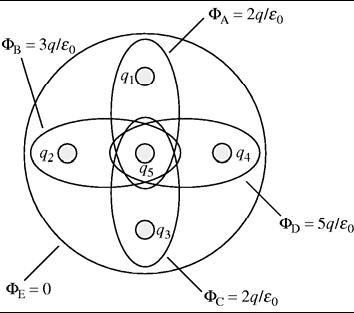# Problem: Five charges are arranged as shown. The figure shows five Gaussian surfaces and the electric flux through each. What are the five charges q1 to q5 in terms of q?

###### FREE Expert Solution

Electric flux:

$\overline{){{\mathbf{\varphi }}}_{{\mathbf{E}}}{\mathbf{=}}\frac{{\mathbf{Q}}_{\mathbf{enc}}}{{\mathbf{\epsilon }}_{\mathbf{0}}}}$, where Q is the electric charge in the given cross-sectional area and ε0 is the permittivity in free space.

From  our figure, $\begin{array}{rcl}{\mathbf{\varphi }}_{\mathbf{A}}& \mathbf{=}& \frac{\mathbf{2}\mathbf{q}}{{\mathbf{\epsilon }}_{\mathbf{0}}}\end{array}$

From the equation of electric flux,

$\begin{array}{rcl}\frac{{\mathbf{Q}}_{\mathbf{e}\mathbf{n}\mathbf{c}}}{{\mathbf{\epsilon }}_{\mathbf{0}}}& \mathbf{=}& \frac{\mathbf{2}\mathbf{q}}{{\mathbf{\epsilon }}_{\mathbf{0}}}\\ {\mathbf{Q}}_{\mathbf{e}\mathbf{n}\mathbf{c}}& \mathbf{=}& \mathbf{2}\mathbf{q}\end{array}$

The charge enclosed by the Gaussian surface, A is:

Qenc = q1 + q5 = 2q

q1 = 2q - q5 ........(a)

98% (472 ratings)###### Problem Details

Five charges are arranged as shown. The figure shows five Gaussian surfaces and the electric flux through each. What are the five charges q1 to q5 in terms of q?Frequently Asked Questions

What scientific concept do you need to know in order to solve this problem?

Our tutors have indicated that to solve this problem you will need to apply the Gauss' Law concept. You can view video lessons to learn Gauss' Law. Or if you need more Gauss' Law practice, you can also practice Gauss' Law practice problems.